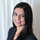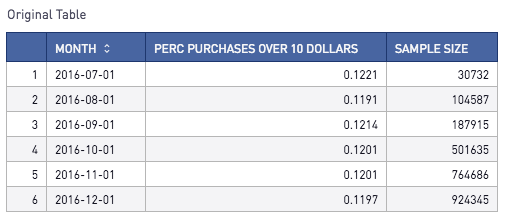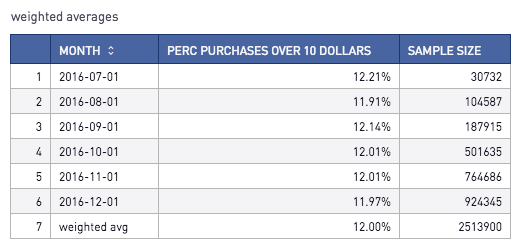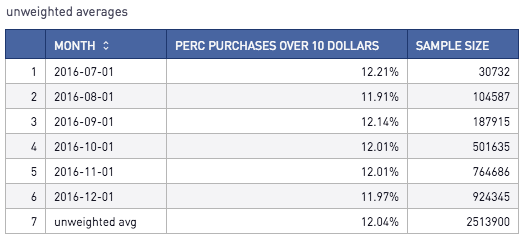# Weighted vs Unweighted Averages

When summarizing statistics across multiple categories, analysts often have to decide between using weighted and unweighted averages.

An unweighted average is essentially your familiar method of taking the mean. Let's say 0% of users logged into my site on Day 1, and 100% of users logged in on Day 2. The unweighted average for the 2 days combined would be (0% + 100%)/2 = 50%.

Weighted averages take the sample size into consideration. Let's say in the example above, there was only 1 user enrolled on Day 1 and 4 users enrolled on Day 2 - making a total of 5 users over the 2 days. The weighted average is 0% * (1/5) + 100% * (4/5) = 80%. Typically, users want to calculate weighted averages as it prevents skewing from categories with smaller sample sizes.

If we want to add a row with a weighted average, we can accomplish this via SQL as shown in the example below (note that this example leverages Redshift syntax).

Let's walk through an example of how to calculate each of these measures! Let's say our original table, t1, contains the following data:Here is how to calculate the weighted average. To add an extra 'Total' row, I used a SQL Union all.

```select
month::varchar(12)
, (round(perc_purchases_over_10_dollars * 100, 2) || '%') as perc_purchases_over_10_dollars
, sample_size
from
t1
union all
select
'weighted avg'
, (round(sum(perc_purchases_over_10_dollars * sample_size) / sum(sample_size) * 100, 2) || '%')
, sum(sample_size)
from
t1
group by
1```

The result looks like this:On the contrary, if we would prefer to use an unweighted average, we can simply union an avg() of each of the categories.  (The additional round/decimal casting is for formatting purposes.)

```select
month::varchar(15)
, (round(perc_purchases_over_10_dollars * 100, 2) || '%') as perc_purchases_over_10_dollars
, sample_size
from
t1
union all
select
'unweighted avg'
, (round(avg(perc_purchases_over_10_dollars) * 100, 2)::decimal(6,2) || '%')
, sum(sample_size) as sample_size
from
t1```

Here, we can see that the results differ from the weighted average example (12.04% as opposed to 12.00%).Note that unweighted and weighted averages are equal if each category has the same sample size.

***Interested in learning more about adding a 'Total' Row or Column? Check out our community post here.***

Reply Oldest first
• Oldest first
• Newest first
• Active threads
• PopularLike Follow
• 9 mths agoLast active
• 1376Views
• 1 Following